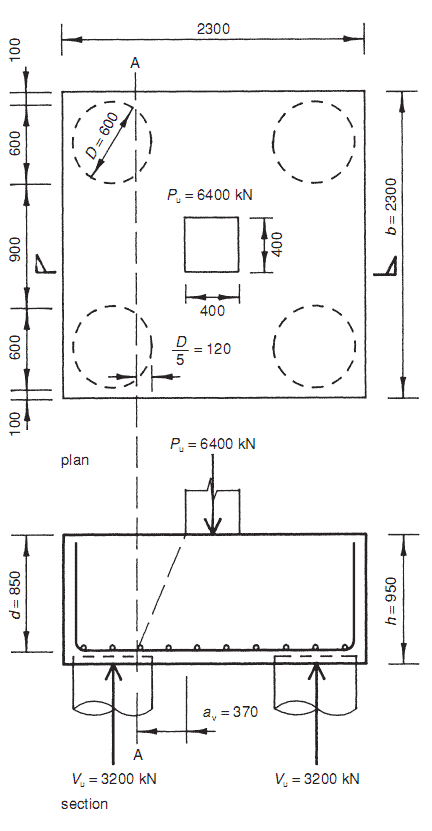### Example: Pile cap design.

A pile cap is required to transfer the load from a 400 mm × 400 mm column to four 600 mm diameter piles, as shown in Fig. 14.30.

Pile caps can be designed either by the truss analogy or by bending theory (see BS 8110: Part 1: 3.11.4.1(5)). In this example bending theory will be used.

For a pile cap with closely spaced piles, in addition to bending and bond stress checks, a check should be made on the local shear stress at the face of the column, and a beam shear check for shear across the width of the pile cap. For more widely spaced piles (spacing  > 3  × diameter), a punching shear check should also be carried out.

Local shear check
The ultimate column load is Pu = 6400 kN.

Length of column perimeter is u = 2(400 + 400) = 1600 mm.

The shear stress at the face of the column is

Bending shear check
In accordance with BS 8110: Part 1: 3.11.4.3, shear is checked across a section 20% of the diameter of the pile (i.e. D/5) inside the face of the pile. This is section A–A in Fig. 14.30.

The shear force across this section – ignoring the self-weight of the pile cap, which is small in comparison – is given by

The corresponding shear stress is given by  vu = Vu/bvd, where bv is the breadth of section for reinforcement design.

In accordance with BS 8110: Part 1: 3.11.4.4, this must not exceed (2d/av)vc where  av is deﬁned in Fig. 14.30 and  vc is the design concrete shear stress from BS 8110: Part 1: Table 3.8. Thus

For grade C35 concrete, from BS 8110: Part 1: Table 3.8, assuming six T25 bars, the minimum value of  vc is  0.4 N/mm2, giving

Thus, provided the average effective depth exceeds  d = 846 mm (the local shear check), minimum reinforcement  to satisfy bond and bending tension requirements will be adequate in this instance.

The necessary depth for the pile cap is
h = d + 25(diameter bar) + 75(cover)
= 846 + 100
= 946 mm ⇒use h = 950 mmFig. 14.30 Pile cap design example.

#### 7 comentarios:

mandiri rahayu sentosa said...

waw complicated calculations also about pilling

Awg Afizal said...

Needed this, Thanks!

Awg Afizal said...

May i ask? Shouldn't the ult column load divided by 4 instead of 2 in the shear force calculation since the column load will be transferred to all four piles?

Unknown said...

how you got av please describe inn details

intan intan said...

Good day. I have a question. By referring to Figure 14.30, how do you find the av? I appreciate if you could answer my question. Thank you :)

Unknown said...

Hi - In this example av is calculated as below:
av = (900 - 400)/2 + (600/5) = 370mm
However, this sketch appears to be wrong as minimum spacing between piles should be 3* Pile Dia. = 3*600 = 1800mm

If that was the case then av can be calculated as:

av = (2*Pile Dia. - Column width)/2 + (Pile Dia/5)